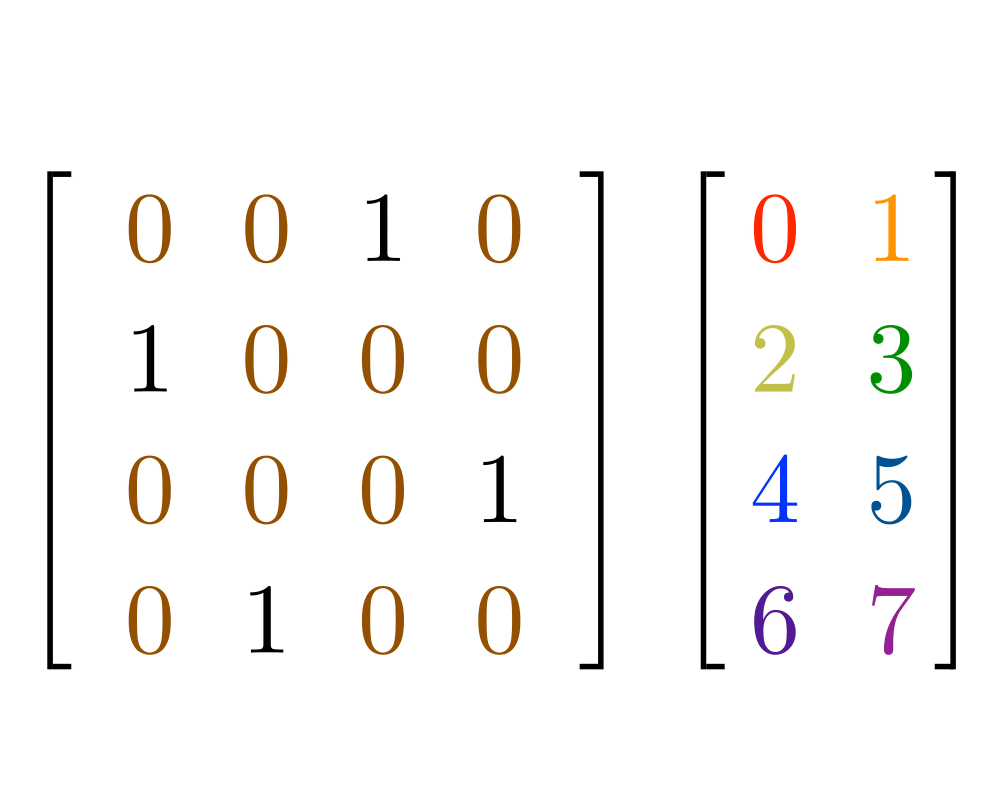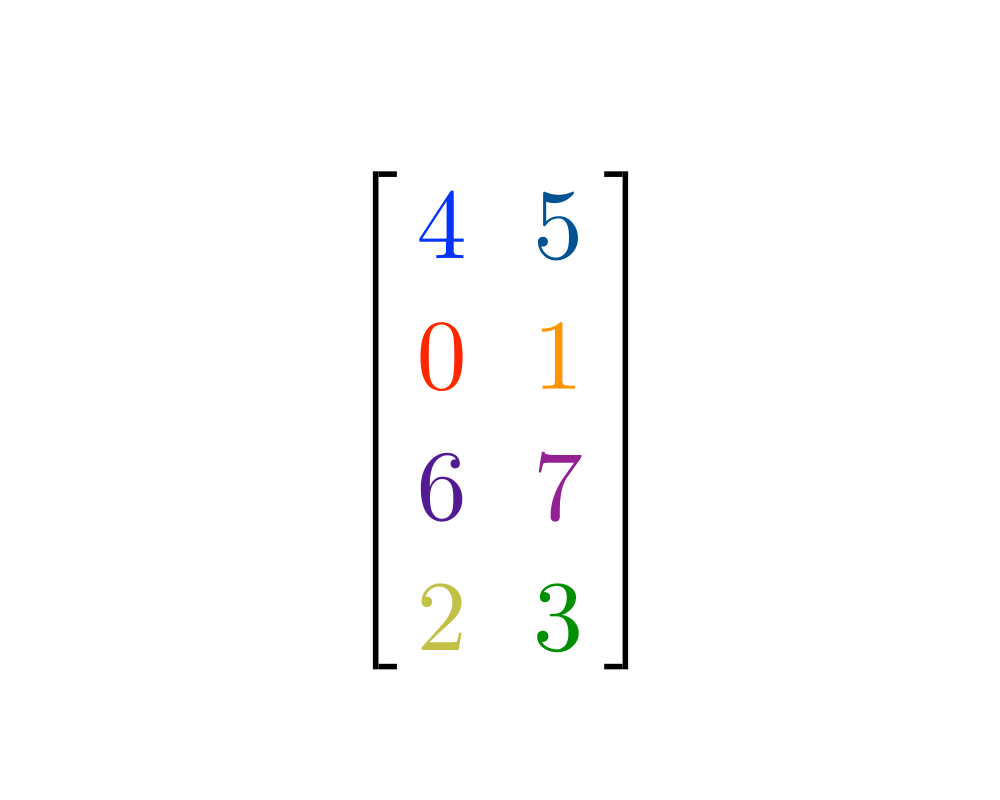# Matrix MultiplicationInputOutput

Input Description: An $$x x y$$ matrix $$A$$, and an $$y x z$$ matrix $$B$$.
Problem: The $$x x z$$ matrix $$A x B$$.

Excerpt from The Algorithm Design Manual: Although matrix multiplication is an important problem in linear algebra, its main significance for combinatorial algorithms is its equivalence to a variety of other problems, such as transitive closure and reduction, solving linear systems, and matrix inversion. Thus a faster algorithm for matrix multiplication implies faster algorithms for all of these problems. Matrix multiplication arises in its own right in computing the results of such coordinate transformations as scaling, rotation, and translation for robotics and computer graphics.

Asymptotically faster algorithms for matrix multiplication exist, based on clever divide-and-conquer recurrences. However, these prove difficult to program and require very large matrices to beat the trivial algorithm.

Go To Main Page